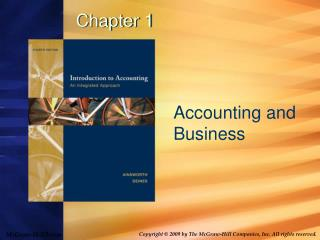Download PresentationChapter 1

# Chapter 1 - PowerPoint PPT PresentationDownload Presentation## Chapter 1

- - - - - - - - - - - - - - - - - - - - - - - - - - - E N D - - - - - - - - - - - - - - - - - - - - - - - - - - -
##### Presentation Transcript

1. Chapter 1 Accounting and Business

2. Bell Ringer……… • Pick up and complete vocabulary matching worksheet. • Pick up a copy of Formula Guide and outline. • Pick up a vocabulary flashcard and stand under the appropriate financial statement poster that relates to your flash card.

3. Essential Questions • How does financial ratios help users of financial statements? Explain.

4. Students will be able to: • Explain the purpose of financial ratios. • Define and calculate the current ratio and debt-equity ratio. • Define and calculate return on sales (net profit margin) and return on equity. • Examine and discuss calculated financial ratios.

5. What are the Purposes of the Ratios? How do they help users of financial statements? • Current ratio - • Relationship between current assets and current liabilities • Measures Liquidity and reflects company’s ability to satisfy its current obligations/ debt. CURRENT ASSETS CURRENT LIABILITIES • Example: Apple 2008 \$34,690 = 2.46 \$ 14,092 Apple had 2.46 of current assets for every \$1.00 of current liabilities

6. What are the Purposes of the Ratios? How do they help users of financial statements? • Return on sales • Relationship between net income and sales; measure of profitability (ability to generate profit from sales) NET INCOME SALES Example: Apple \$4,834 = 14.88% 32,479 Apple generated 14.88% profit per \$1 sales or .15 of every \$1 is profit.

7. What are the Purposes of the Ratios? How do they help users of financial statements? • Debt to equity ratio • Relationship between liabilities and owners’ equity; measure of company solvency (ability to pay both short- and long-term obligations) • More debt a company has, more profits it must generate to pay the interest on debt and the greater risk for shareholders. TOTAL DEBT TOTAL SHAREHOLDERS’ EQUITY

8. What are the Purposes of the Ratios? How do they help users of financial statements? • Debt to equity ratio TOTAL DEBT TOTAL SHAREHOLDERS’ EQUITY • Example: Apple \$18,542 = \$.88 21,030 • Apple had .88 of current and long-term debt for every \$1.00 of shareholders’ equity. • Ratio 1 to 1 = half company’s assets are financed with debt and half with shareholders’ equity.

9. Lecture Examples 3. Using the amounts shown in Lecture Example #2, calculate the current ratio, the debt to equity ratio, and the return on sales ratio. Answer: Current ratio: Debt to equity ratio: Return on sales ratio:

10. Lecture Examples 2. Describe each of the following items and determine which financial statement it appears on. Accounts payable, \$136 Building, \$809 Accounts receivable, \$876 Patent, \$2 Cash received from customers, \$13,074 Long-term bank loan, \$716 Cash paid for inventory, \$8,338 Common stock, \$3,827 Cash paid to employees, \$1,724 Retained earnings, \$373 Cost of goods sold, \$8,192 Cash balance, \$2,211 Inventory, \$908 Miscellaneous payables, \$529 Miscellaneous operating expenses, \$3,686 Sales, \$13,353 Wage expense, \$1,750

11. Essential Questions • How does financial ratios help users of financial statements? Explain.

12. Students will be able to: • Explain the purpose of financial ratios. • Define and calculate the current ratio and debt-equity ratio. • Define and calculate return on sales (net profit margin) and return on equity. • Examine and discuss calculated financial ratios.

13. HOMEWORK……. • Finish final section of skeletal outline page 6. • Complete E1.9 & 1.17 • Complete Case 1A • THANK YOU!!!!!!